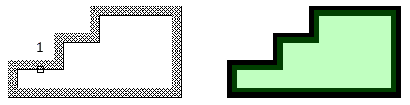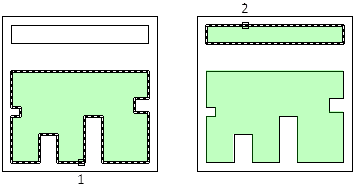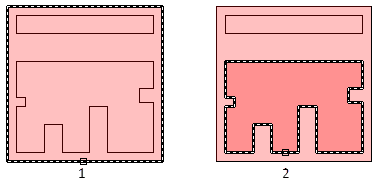# AREA (Command)

Calculates the area and perimeter of objects or of defined areas.

You can obtain measurements by selecting an object, or by specifying points to define what you want to measure. The area and perimeter of the specified object are displayed at the Command prompt and in the tooltip.

The MEASUREGEOM command provides additional measurement options. You can also use BOUNDARY to create a closed polyline or region and then use LIST or the Properties palette to find the area.

The following prompts are displayed.

### Specify first corner point

Calculates the area and perimeter defined by specified points. Start by specifying the first point. All points must lie in a plane parallel to the XY plane of the current user coordinate system (UCS).

Specify next point
A rubberband line from the first specified point to the cursor is displayed. Continue to specify points to define a polygon and then press Enter to complete the definition of the perimeter. The area to be calculated is highlighted in green.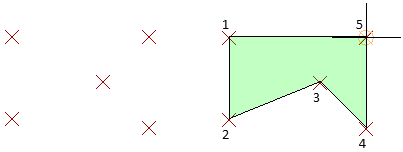If you do not close the polygon, the area is calculated as if a line were drawn from the last point entered to the first. When the perimeter is calculated, that line length is added.

### Object

Calculates the area and perimeter of a selected object.

Select objects

Select objects such as circles, ellipses, splines, polylines, polygons, regions, and 3D solids.

If you select an open polyline, the area is calculated as if a line were drawn from the last point entered to the first. When the perimeter is calculated that line length is ignored.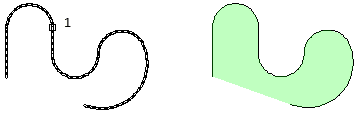The centerline of a wide polyline is used to make area and perimeter (or length) calculations.